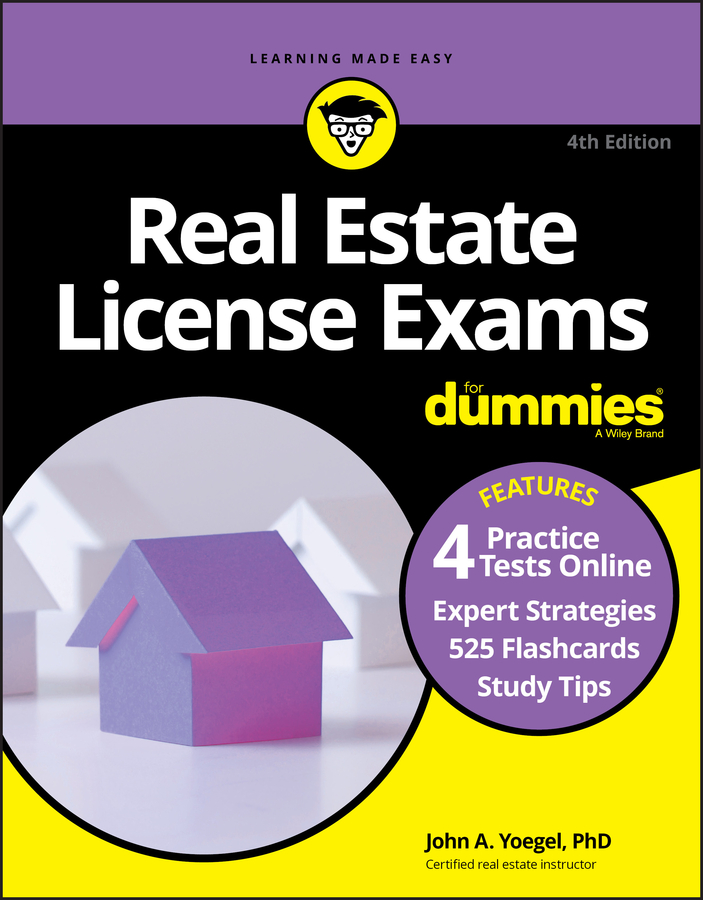##### Real Estate License Exams For Dummies with Online Practice TestsAs a real estate agent, you won’t be doing appraisals, but you will use these formulas to estimate property values for the Real Estate License Exam. The only real math-driven formulas for appraising, at least at the level that you’re expected to know it, are formulas for finding value using the income approach. Two different methods are used in that approach to value. Here are formulas and examples for both.

## The capitalization method

The capitalization method uses one formula for finding a property’s value, and with a couple of variations you can find the property’s income and the capitalization rate. Capitalization is a technique for estimating a property’s value based on its income.

The formulas use the following symbols:

• I = Income or net operating income

• R = Rate of return or capitalization rate

• V = Value or sale price

Don’t be confused by the possibility that any one of these letters can mean two different things (such as income versus net operating income). Memorize these terms and the problem will be clear as to what is being asked. Also remember that in these problems, all the numbers are based on annual figures. So if, for example, you’re given a monthly income number, you need to multiply by 12.

The formulas follow:

• V = I ÷R

• I = V x R

• R = I ÷V

Find the value of a building that has a net operating income of \$30,000 where the capitalization rate is 10 percent.

V = \$30,000 ÷0.10

V = \$300,000

Find the income of a building that was sold \$300,000 where the rate of return is 10 percent.

I = \$300,000 x 0.10

I = \$30,000

Find the capitalization rate of a building that sold for \$300,000 and has a net operating income of \$30,000.

R = \$30,000 ÷\$300,000

R = 0.10 or 10 percent

## The gross rent multiplier method

The method for finding the value of a property using the gross rent is an easy matter of multiplication. The gross rent multiplier is used to estimate the value of small investment-type properties like small multifamily houses. The formula is

Value = rent x gross rent multiplier (GRM)

The variations of this equation are

GRM = sale price (or value) ÷rent

Rent = sales price (or value) ÷GRM

The following examples show you how to use these formulas with numbers.

You’re appraising a building that generates a gross annual rent of \$36,000. You’ve calculated a GRM of 10. What is the value of the building?

Value = \$36,000 x 10

Value = \$360,000

You’re trying to calculate GRM using information on a building that recently sold for \$360,000 with a gross annual rent of \$36,000. Find the GRM.

GRM = \$360,000 ÷ \$36,000

GRM = 10

You would rarely have to calculate the rent using the GRM formula, but just in case, here’s one last example.

Say you were looking at a building to invest in that was selling for \$360,000 with a GRM of 10. What is the annual gross rent income?

Rent = \$360,000 ÷10

Rent = \$36,000

Don’t forget to note whether the numbers you’re given are based on monthly rent or annual rent. You may have to convert one to the other or use the number given. Gross rent multipliers are sometimes calculated on an annual basis and are sometimes called the Gross Income Multipliers (GIM). Your course should clarify the terminology used for your exam.